Sunday , January 17 2021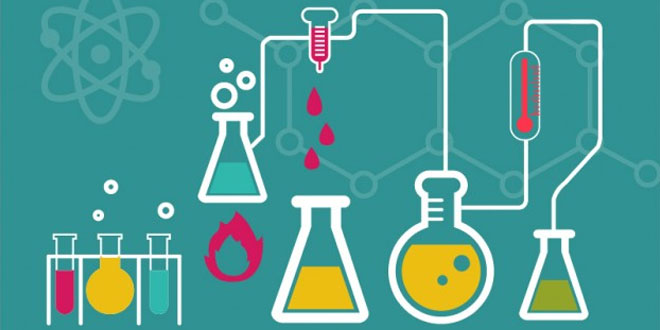# 10 CBSE Science Paper Design (2013-14) Outside Delhi

## 10 CBSE Science Paper Design (2013-14) Outside Delhi

#### Modified Based on Latest CBSE Question Paper Design

Time allowed: 3 hours
Maximum marks: 80
Class: X
Subject: Science

Year: 2013 – 14 (Outside Delhi)

## General Instructions:

• The Question paper comprises of two sections, A and B. You are to attempt both the sections.
• All questions compulsory.
• All questions of Section A and all questions of Section B are to be attempted separately.
• Question number 1 to 2 in Section A are one mark questions. These are to be answered in one word or in one sentence.
• Question numbers 3 to 5 in Section A are two marks questions. These are to be answered in about 30 words each.
• Question numbers 6 to 15 in Section A are three marks questions. These are to be answered in about 50 words each.
• Question numbers 16 to 21 in Section A are five marks questions. These are to be answered in about 70 words each.
• Question numbers 22 to 27 in Section B are questions based on practical skills and are two marks question.

## Section A

#### Question: 1. Write the relation between electric power (W) of a device with potential difference (Volt) across it and current (amp) flowing through it. 

Answer: The power (p) in watts is found by multiplying the potential different (V) in volts by the current (I) in amperes.
Electric power = Potential difference × Current
P = V × I

#### Question: 2. What is gene? 

Answer: A gene is a unit of DNA on a chromosome which governs the synthesis of one protein that controls a specific character of a organism. Thus genes are actually units of heredity which transfer characteristics from parents to their offspring reproduction.

#### Question: 3. What is the difference between sperms and eggs of humans? Write the importance of this difference. 

Answer: The main difference between sperms and eggs of humans is that half of the sperms of a male (father) will have X chromosomes and the other half will have Y chromosomes. All the eggs of female (mother) will have only X chromosomes.
Importance: If the father contributes X sex chromosome, at fertilization through his sperm, the baby born will be a girl. On the other hand, if the father contributes a Y sex chromosome at fertilization through his sperm, then the baby born will be a boy. Therefor the sperm determines the sex of the child.

#### Question: 4. Give reason for the following: (a) Sodium metal is kept immersed in kerosene. (b) Blue colour of copper sulphate solution disappears when some aluminium power is added in it. 

Answer: (a) Sodium metal is so reactive that it reacts vigorously with the oxygen (of air). It catches fire and stars burning when kept open in the air. Sodium metal is stored under kerosene oil to prevent its reaction with oxygen, moisture and carbon dioxide of air.
(b) When aluminium powder is put in copper sulphate solution, then the blue colour of copper sulphate solution fades gradually due to the formation of colorless aluminium sulphate solution.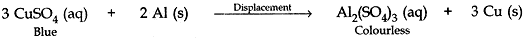Since Al is more reactive than Cu, it displaces Cu from CuSO4 solution and forms colorless Al2(SO4)3.

#### Question: 5. Give two reasons why different electrical appliance in a domestic circuit are connected in parallel. 

Answer: The  arrangement of lights and various other electrical appliances in parallel circuits is used in the domestic wiring because of following advantages:

1. In parallel circuits, if one electrical appliance stops working due to some defects, then all other appliances keep working normally.
2. In parallel circuits each electrical appliance has its own switch due to which it can be turned on or turned off independently, without affecting other appliances.

#### Question: 6. Solid calcium oxide was taken in a container and water was added slowly to it. (i) State two observations made in the experiment. (ii) Write the balanced chemical equation of this reaction. 

Answer: (i) Solid calcium oxide was taken in a container and water was added to it.
Observations:
(a) The mixture produces a hissing sound.
(b) The beaker feels to be quite hot.
(ii)        CaO (s)           +        H2O           →            Ca(OH)2             +             Heat
calcium oxide(lime)                                             calcium hydroxide(slaked flame)

#### Or Write balanced chemical equations for the following reactions: (a) dilute sulphuric acid reacts with aluminium powder. (b) dilute hydroelectric acid reacts with sodium carbonate. (c) Carbon dioxide is passed through lime water.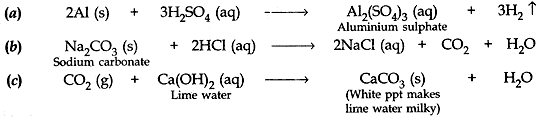#### Question: 7. Explain the effect on the magnetic field produced at a point in a current carrying circular coil due to: (1) increase in the amount of current flowing through it (2) increase in the distance of point from the coil (3) increase in the number of turn of the coil. 

1. The magnitude of field produced by a current carrying circular coil at a point is directly proportional to the current flowing through the circular coil. Thus as the amount of current flowing through the circular coil increases, the magnetic field produced at a point in a circular coil increases.
2. Magnitude of magnetic field produced at a point in a current carrying circular coil is inversely proportional to the distance increases from the coil.
3. The magnetic field produced by a current carrying wire at a given point depends directly on the current passing through it. Therefore, if there is a circular coil having n turns, the field produced is n times as large as that produced by a single turn. This is because the current in each circular turn has the same direction, and the field due to such turns then just adds up.

#### Question: 8. An electric lamp and a conductor of resistance 4Ω are connected in series to a 6 V battery. The current drawn by the lamp is 0.25A. Find the resistance of the electric lamp.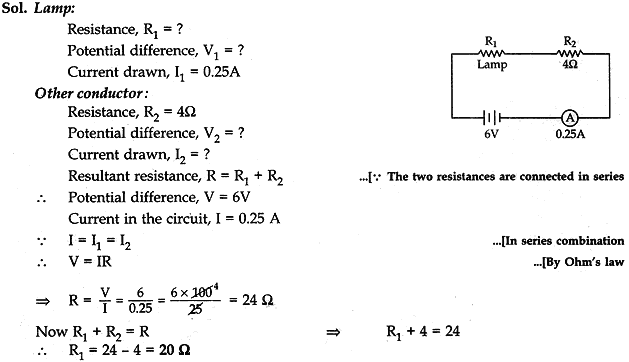#### Question: 9. Define the following: (1) Reflex action    (2) Synapse     (3) Phototropism  

1. Reflex action: A reflex action defined as a spontaneous, automatic and mechanical response to a stimuli without the will of a living being. In such actions there is no involvement of the brain. All reflex actions are conveyed through the spinal cord by a path called reflex arc.
2. Synapse: A microscopic gap between a pair of adjacent neurons over which nerve impulses pass when going from one neuron to the next is called a synapse.
3. Phototropism: It is the response of the plant parts to the external stimulus of light. The stem shows positive phototropism as the stem of the plant grows in the direction of light while root shows negative phototropism as the plant grows away from the light. This growth is controlled by the auxin hormone.

#### Question: 10. What do you mean by bio magnification of harmful chemicals? How does this phenomenon affect the human health? 

Answer: Bio magnification of harmful chemicals: Harmful chemicals enter through the soil and water in the first trophic level of the food chain and then their concentration goes on increasing in the second trophic level to the last trophic level.
This increasing concentration of toxic substances within each successive trophic level in the food chain is known as bio magnification of harmful chemicals.
Human being is at the last trophic level of its food chain. So harmful chemicals enter into humans bodies in maximum quantity and thus damage our internal body organs and cause many types of diseases in our body.

#### Question: 11. What are homologous series of carbon compounds? Write the molecular formula of two consecutive members of homologous series of aldehydes. State which part of these compounds determines their (i) physical and (ii) chemical properties. 

Answer: Homologous series: A series of carbon compounds in which the same functional group substitutes for hydrogen on a carbon chain is called a homologous series. There is a difference of -CH2 in the molecular formulae of two nearest compounds of a homologous series. Each such series has same general molecular formula and has a general scientific name. There is a difference of 14 μ (unified mass) in the molecular masses of two nearest compounds of a series.
Members of homologous series of aldehydes:
H       – CHO          Methanal
CH3   – CHO         Ethanal
C2H5 – CHO        Propanal
(i) The carbon-chain attached to aldehyde group, i.e., (H –, CH3 –,  C2H5 –) determine the physical properties.
(ii) The functional group attached to all aldehydes (– CHO) determines the chemical properties.

#### Question: 12. Study the following table in which positions of six elements A, B, C, D, E and F are shown as they are in the modern periodic table: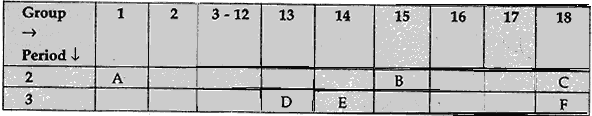#### On the basis of the above table, answer the following questions: (1) Name the element which forms only covalent compounds. (2) Name the element which is a metal with valency three. (3) Name the element which is a non-metal with valency three. (4) Name the element which is bigger in size and why? (5) Write the common name for the family to which the elements C and F belong.

1. E
2. D
3. B
4. D is bigger in size because as we move from left to right in a period, the size of the atom decreases as positive charge increases but the number of shells remains the same. Thus the electrons are pulled in more close to the nucleus.
5. Noble gases

#### Question: 13. With the help of suitable examples, explain why certain traits cannot be passed on to the next generation. What are such traits called? 

Answer: A trait of an organism which develops in response to the environment and brought in the non-reproductive tissues can not be passed on to the DNA of the germ cells. Such traits are called acquired traits. An individual cannot pass these traits to its progeny as these traits cannot be passed on to the DNA of the germ cell.
Example:
(i) If a beetle does not get sufficient food for a considerable time, its weight will reduce due to starvation. The low weight is an acquired trait.
(ii) Cut tail of a mouse.

#### Or “A trait may inherited, but may not expressed.” Justify this statement with the help of suitable example. 

1. Mendel crossed pure-bred tall pea plants with pure-bred dwarf pea plants and found that only tall pea plants were produced in the Fgeneration.
2. Mendel then crossed the tall pea plants of Fgeneration and found that tall plants and dwarf plants were obtained in the Fgeneration in the ratio of 3:1.
3. This experiment shows that the traits of dwarfness had not been lost but it was merely concealed in the F1 generation.

Thus “A trait may be inherited, but may not be expressed.”

#### Question: 14. A student wants to project the image of  a candle flame on a screen 60 cm in front of a mirror by keeping the flame at a distance of 15 cm from its pole.  (i) Write the type of mirror he should use. (ii) Find the liner magnification of the image produced. (iii) What is the distance between the object and its image? (iv) Draw a ray diagram to show the image formation  in this case.

(ii) Linear magnification = – Image distance/Object distance
m = –v/u
Object distance, u = -15 (u is always negative)
Image distance, v = -60 (v is negative for real image)
m = – (-60)/(-15)
∴       m = – 4
The minus sign in magnification shows that the image formed is real and inverted.
(iii) Distance between object and its image = 45 cm
(iv) Ray diagram: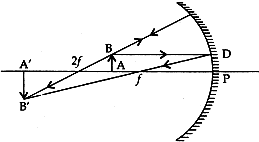The image formed is real, inverted, magnified and beyond 2f.

#### Question: 15. A narrow beam PQ of white light is passing through a glass prism ABC as shows in the diagram.  (i) Write the name and cause of the phenomenon observed. (ii) Where else in nature is this phenomenon observed? (iii) Based on this observation, state the conclusion which can be drawn about the constituents of white light.

Answer: (i) The phenomenon of splitting of white light into seven colours on passing through a glass-prism is called dispersion of light. The dispersion of while light occurs because colours of white light travel at different speeds through the glass prism.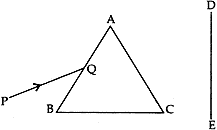(ii) Formation of rainbow is an example of dispersion of white light in nature.
(iii) The formation of spectrum of seven colours shows that white light is made up of lights of seven different colours mixed together. That is, white light is a mixture of seven colours (or seven coloured lights), i.e., red, orange, yellow, green, blue, indigo and violet.#### Question: 16. You are provided with a container made up aluminium. You are also provided with solution of dil HCl, dil HNO3, ZnCl2 and H2O. Our of these solutions which solution, can be kept in the aluminium container? Name the type of reaction taking place. 

Answer: (i) Dilute HCl cannot be kept in aluminium container because aluminium metal reacts rapidly with dil HCl to form aluminium chloride and liberating hydrogen gas.

2Al (s)    +      6HCl (dil)            →               2AlCl(aq)             +       3H(g)
(Displacement reaction)

(ii) When dilute HNOis kept in aluminium container, it forms a protective layer of aluminium oxide on it which makes it passive towards action with acid and therefore, it can be kept in Aluminium container.

2Al (s)    +      6HNO3           →              2Al2O3             +         6NO2          +      3H2O
(Displacement reaction)

(iii) ZnCl2  solution also cannot be kept in aluminium container because  Al is more reactive than Zn, therefore more reactive Al displaces less reactive Zn from its solution (ZnCl2).

2Al (s)     +      3ZnCl(aq)                  →                  2AlCl3            +        3Zn
(Displacement reaction)

(iv) Aluminium does not react with H2O. So, can be kept in aluminium container.

#### Question: 17. (a) Two identical resistors each of resistance 10 ohm are connected: (i) in series (ii) in parallel, in turn to a battery of 6V. Calculate the ratio of power consumed in the combination of resistors in the two cases. (b) Establish the relationship between 1 kWh and SI unit of energy.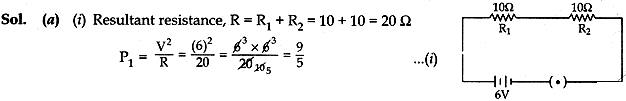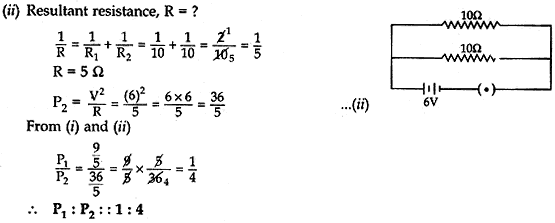(b) Relation between kilowatt-hour and joule (SI unit of energy):
1 kilowatt-hour is the amount of energy consumed at the rate of 1 kilowatt for 1 hour.
i.e., 1 kilowatt-hour = 1 kilowatt for 1 hour
or    1 kilowatt-hour = 1000 watts for 1 hour#### Question: 18. (1) Compare the length of small intestine in herbivore and carnivore animal. (2) Mention any two structural modifications in small intestine which helps in absorption. 

1. The length of the small intestine differs in various animals depending on the type of food they eat. For example, cellulose is a carbohydrate food which is digested with difficulty. So, the herbivorous animals like cow which eat grass need a longer ‘small intestine’ to allow the cellulose present in grass to be digested completely. On the other hand, meat is a food which is easier to digest. So, the carnivorous animals like tigers which eat meat, have a shorter ‘small intestine’.
2. The inner surface of small intestine has millions of tiny, finger-like projections called villi. The presence of villi gives the inner walls of the small intestine a very large surface area and the large surface area of small intestine in the rapid absorption of digested food.

#### Question: 19.(a) Draw a sectional view of human female reproductive system and label the part where (i) eggs develop. (ii) fertilization take place. (iii) fertilized egg gets implanted. (b) Describe, in brief, the changes the uterus undergoes (i) to receive the zygote. (ii) if zygote is not formed. 

Answer: (a) Sectional view of human female reproductive system: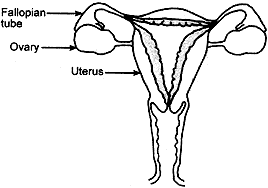Parts where:
(i) eggs develop – Ovary
(ii) fertilization take place – Fallopian tubes
(iii) fertilized egg gets implanted – Uterus

(b) (i) The inner lining of the uterus becomes thick and soft with lots of blood capillaries before release of a fertilised egg (zygote). The uterus has to keep this zygote for further development and supply it with food, oxygen, etc.
(ii) if no fertilisation occurs then the thick uterus lining breaks down along with its bloods vessels in the form of vaginal bleeding called menstrual flow.

#### Question: 20. (a) Explain the following terms related to spherical lenses: (i) Optical centre (ii) Centres of curvature (iii) principal axis (iv) aperture (v) principal focus (vi) focal length (b) A converging lens has focal length of 12 cm. Calculate at what distance should the object be placed from the lens so that it forms an image at 48 cm on the other side of the lens. 

Answer: (a) (i) Optical centre: It is a point within the lens that lies on the principal axis through which a ray of light passes undeflected.
(ii) Centre of curvature: The centre of curvature of the surface of a lens is the centre of the sphere of which it forms a part. A lens has two centres of curvature because it has two surfaces.
(iii) Principal axis: It is a line through the centres of curvature of the lens.
(iv) Aperture: The diameter of the circular boundary of the lens is called the aperture of the lens.
(v) Principal focus: A beam of light parallel to the principal axis either converges to a point or appears to diverge from a point on the principal axis after refraction through the lens, is called the principal focus. All lenses have two principal focuses.
(vi) Focal length: The distance between the optical centre and the principal focus of the lens is called its focal length.
(b) A converging lens is a convex lens.
f = +12 cm         u = ?       v = +48 cm (+ve as it is formed on other side of the object) According to lens formula,

1/f = 1/v – 1/u  ⇒  1/12 = 1/48 – 1/u

1/u = 1/48 – 1/12 = 1-4/48 = -3/48 = 1/16 ∴  u = -16 cm
∴ The object should be placed 16 cm away from the lens.

#### Question: 21. (1) Why do we classify elements? (2) What were the two criteria used by Mendeleev in creating his Periodic Table? (3) Why did Mendeleev leave some gaps in his Periodic Table? (4) In Mendeleev’s Periodic Table, why was there no mention of Noble gases like Helium, Neon and Argon? (5) Would you place the two isotopes of chlorine, Cl-35 and Cl-37 in the same slot because their chemical properties are same? Justify your answer. 

1. As different elements were being discovered, scientists gathered more information about the properties of these elements. It was observed that it was difficult to organise all the information or properties of these elements. So scientists started discovering some pattern in their properties to classify all the known elements to make their study easier.
2. Atomic mass and similarity of chemical properties (compounds formed by elements with oxygen and hydrogen) were the who criteria used by Mendeleev in his Periodic Table.
3. Mendeleev left some gaps in his Periodic Table as he predicted the existence of some elements that had not been discovered at that time.
4. Noble gases like helium, neon, argon etc. were not mentioned in Mendeleev’s Periodic Table because these gases were discovered later as they are very inert and present in extremely low concentrations in our atmosphere. After the discovery of noble gases they could be placed in a new group without disturbing the existing order of the Periodic Table.
5. The two isotopes of chlorine Cl-35 and Cl-37 would be placed in different slots of Mendeleev’s Periodic Table as both have different atomic masses. But the two isotopes have same chemical properties so would also be placed in the same slot. This is a contradiction. Thus position of isotopes of an element is not defined in Mendeleev’s Periodic Table.

## Section B

#### Question: 22. A student performed the experiment of heating ferrous sulphate crystals in a boiling tube. He smelt fumes of a pungent gas and saw colors of ferrous sulphate disappear. (i) Write the chemical formula of the pungent gas. (ii) Why does the colour of crystal disappear? (iii) Identify the nature of this chemical reaction. 

Answer: (i) SO2   → Sulphur dioxide
SO → Sulphur dioxide
(ii) Ferrous sulphate crystals contain 7 molecules water of crystallisation (FeSO4.7H2O). These crystals are green in colour. When these crystals are heated, they first lose 7 molecules of water of crystallisation to form anhydrous ferrous sulphate (FeSO4) which is while in colour.
(iii) It is a thermal decomposition reaction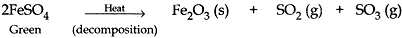because in this reaction one substance is splitting up into three substance on heating.

#### Question: 23. A student prepared a temporary mount of a leaf and observed some green dots. (1) Name these green dots and name pigment in them. (2) State the function of this pigment. 

1. Green dots are chloroplast. it contains green pigment called chlorophyll.
2. Chlorophyll traps solar energy. This solar energy is required by the plant to carry out the chemical reactions involved in the preparation of food (i.e., in photosynthesis).

#### Question: 24. Which is smaller, terminal-p.d. or e.m.f. of a cell and why? 

Answer: Terminal p.d. is smaller. it is because when a cell supplies current, a part of e.m.f. (i.e., some electrical energy) is used in overcoming the internal resistance of the cell. As a result of it. p.d. at the terminals of the cell falls from its maximum value.

#### Question: 24. What happens when acetic acid is added in a solution of Na2CO3 in a test tube? Write the equation for detecting the gas evolved. 

Answer: COgas is evolved with brisk bubbles in this reaction.
White precipitate (ppt.) are formed, when COgas is passed through the lime water.

CO2   + Ca(OH)2(aq)     →      CaCO3(s)   +    H2O
Lime water                          White ppt.

#### Question: 26. When a ray light passes through a glass slab how many times does it change its path and why? 

Answer: The ray of light bends twice.
First time when it enters from air to the glass slab, it bends towards the normal, i.e., from rarer medium to denser medium.
Second time, when the ray moves out from the glass slab to air, it bends away from the normal, i.e., it moves from denser medium to rarer medium.

#### Question: 27. Why do raisins absorb water when soaked in water? Explain the phenomenon. 

Answer: Raisins absorb water due to the process of end-osmosis. 
Water moves from semi permeable membrane of raisins into the raisin, i.e., from hypo-tonic solution to the hyper-tonic solution of raisin cell sap.

## 11th CBSE NCERT Computer Science Mid Term Exam Set A

11th Class CBSE NCERT Computer Science: Mid Term Examination Set – A (2019-20) Time: 3 Hrs. …